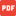Lesson 01 Why believe creation according to Genesis.pdfLesson 02 What is biblical creation and why is it important.pdfLesson 03 Is evolution fact or fiction.pdfLesson 04 Is evolution science.pdfLesson 5 How did man fall.pdfLesson 06 What were the effects of the fall.pdfLesson 07 What is sin.pdfLesson 08 What are the effects of sin.pdfLesson 09 What is man.pdfLesson 10 What are angels.pdfLesson 11 What is the work and fall of angels.pdfLesson 12 Who is Satan.pdfLesson 13 What is the work and destiny of Satan.pdfLesson 14 What are demons (devils).pdfLesson 15 What is the organization and demise of devils.pdfLesson 16 How does Satan attack Christians.pdfLesson 17 How do you overcome Satan.pdfLesson 18 What is faith.pdfLesson 19 How to increase your faith.pdfLesson 20 What is repentance.pdfLesson 21 How do you repent.pdfLesson 22 What is forgiveness.pdfLesson 23 What is forgiveness (2 of 2).pdfLesson 24 What happens when you believe on the Lord.pdfLesson 25 How can you be sure you are saved.pdfLesson 26 What is justification.pdfLesson 27 What is regeneration.pdf